Guides for each scale can be set scale-by-scale with the guide argument, or en masse with guides().

## Usage

guides(...)

## Arguments

...

List of scale name-guide pairs. The guide can either be a string (i.e. "colorbar" or "legend"), or a call to a guide function (i.e. guide_colourbar() or guide_legend()) specifying additional arguments.

## Value

A list containing the mapping between scale and guide.

Other guides: guide_bins(), guide_colourbar(), guide_coloursteps(), guide_legend()

## Examples

# \donttest{
# ggplot object

dat <- data.frame(x = 1:5, y = 1:5, p = 1:5, q = factor(1:5),
r = factor(1:5))
p <-
ggplot(dat, aes(x, y, colour = p, size = q, shape = r)) +
geom_point()

# without guide specification
p
#> Warning: Using size for a discrete variable is not advised.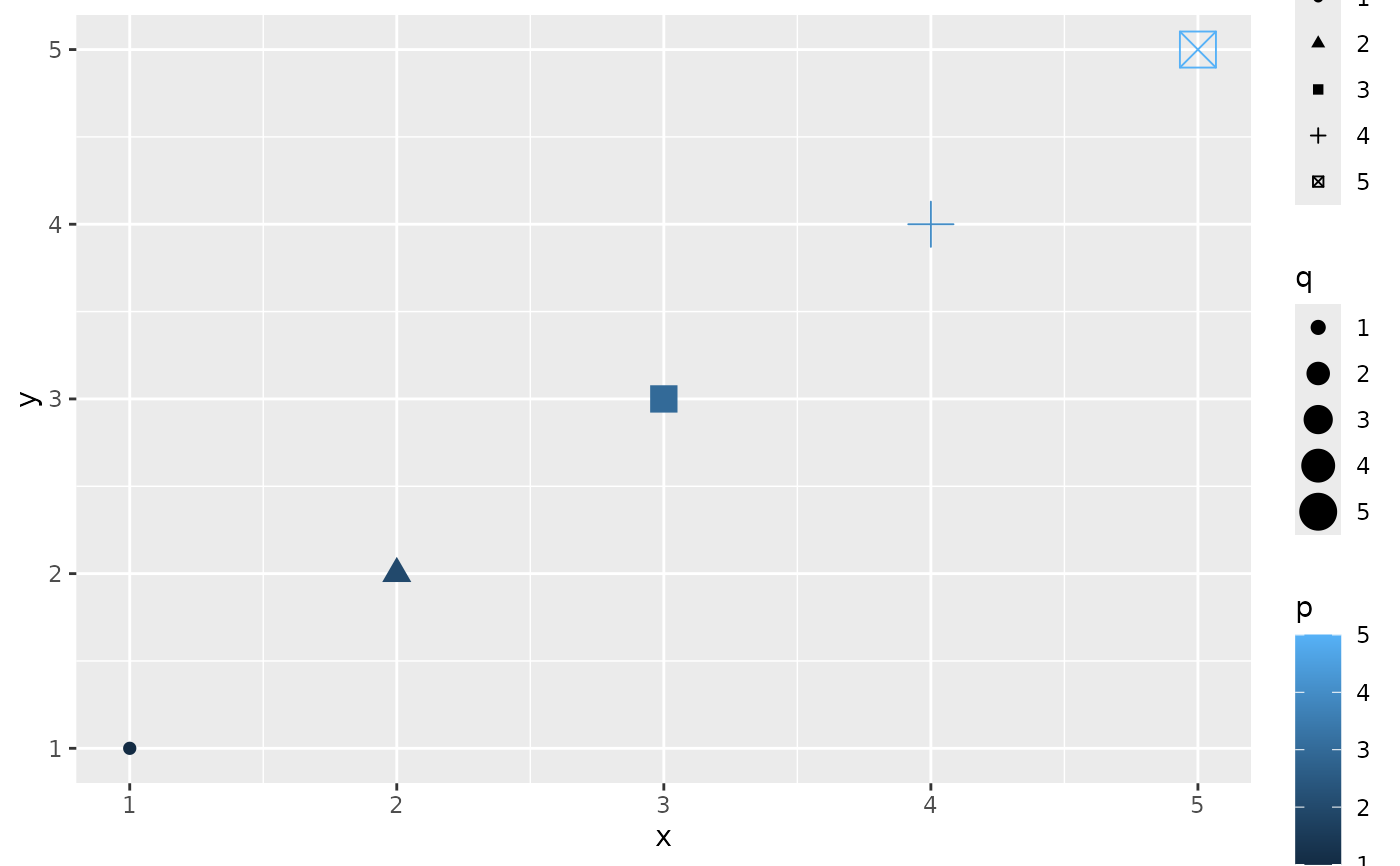# Show colorbar guide for colour.
# All these examples below have a same effect.

p + guides(colour = "colorbar", size = "legend", shape = "legend")
#> Warning: Using size for a discrete variable is not advised.p + guides(colour = guide_colorbar(), size = guide_legend(),
shape = guide_legend())
#> Warning: Using size for a discrete variable is not advised.p +
scale_colour_continuous(guide = "colorbar") +
scale_size_discrete(guide = "legend") +
scale_shape(guide = "legend")
#> Warning: Using size for a discrete variable is not advised.# Remove some guides
p + guides(colour = "none")
#> Warning: Using size for a discrete variable is not advised.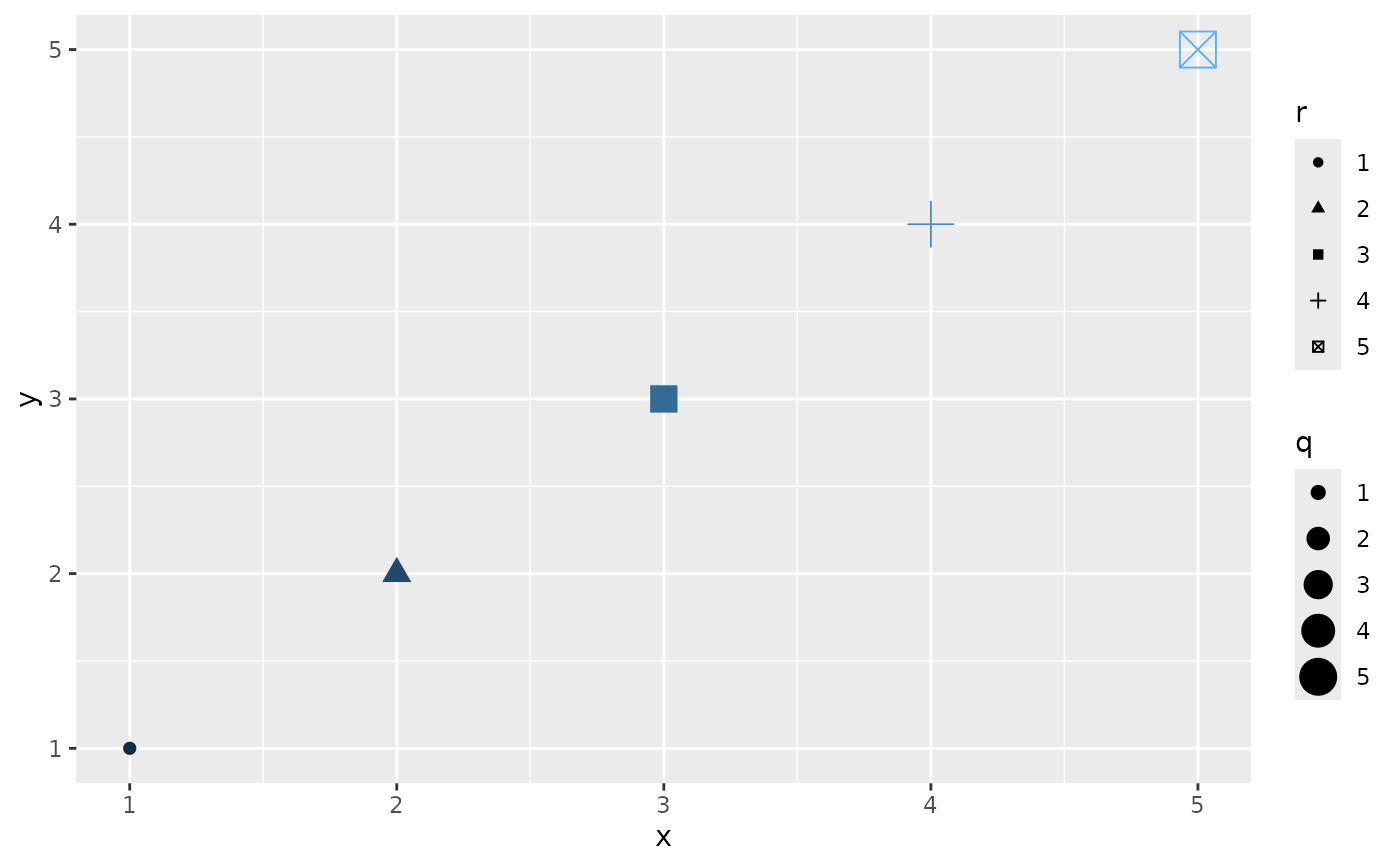p + guides(colour = "colorbar",size = "none")
#> Warning: Using size for a discrete variable is not advised.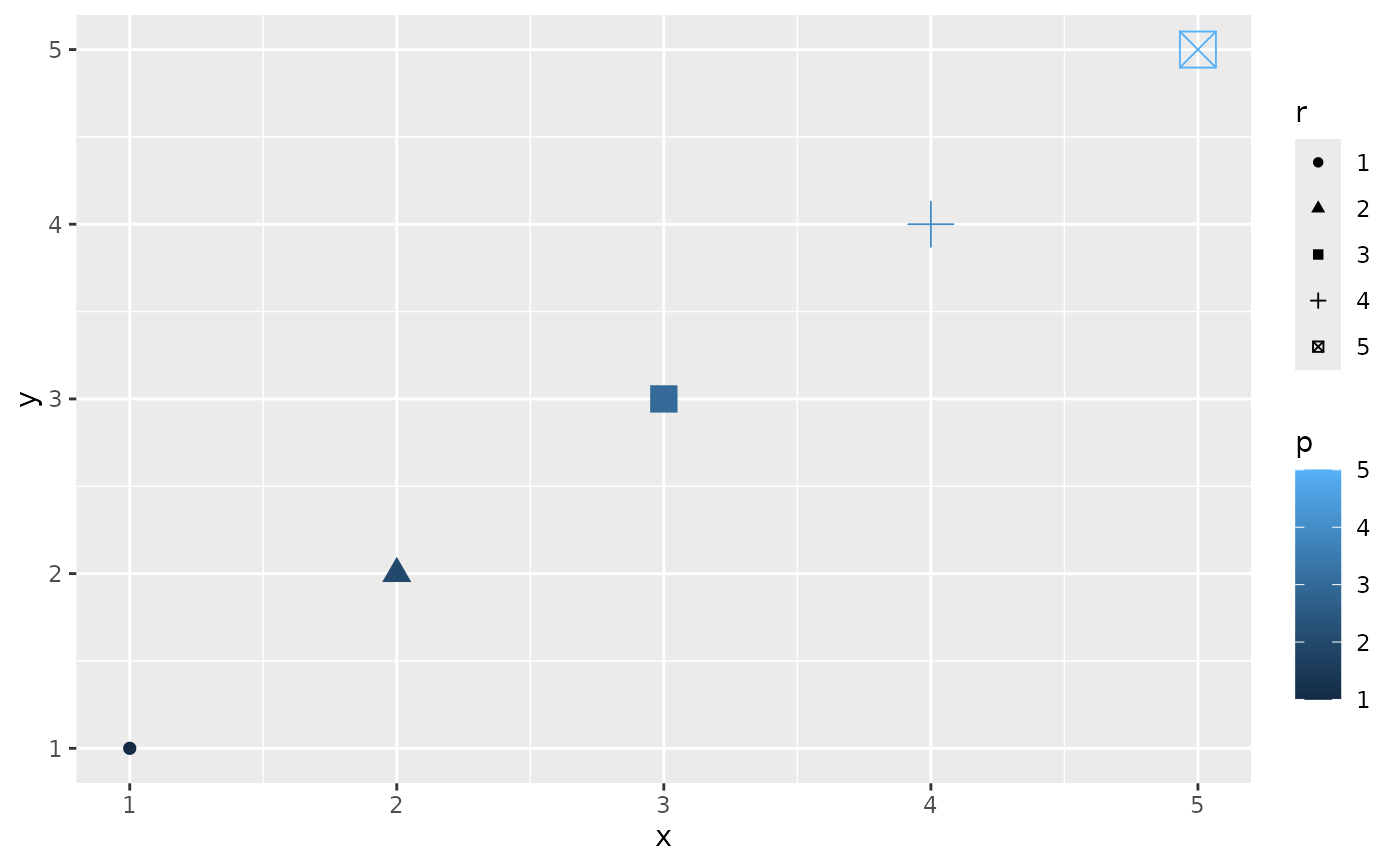# Guides are integrated where possible

p +
guides(
colour = guide_legend("title"),
size = guide_legend("title"),
shape = guide_legend("title")
)
#> Warning: Using size for a discrete variable is not advised.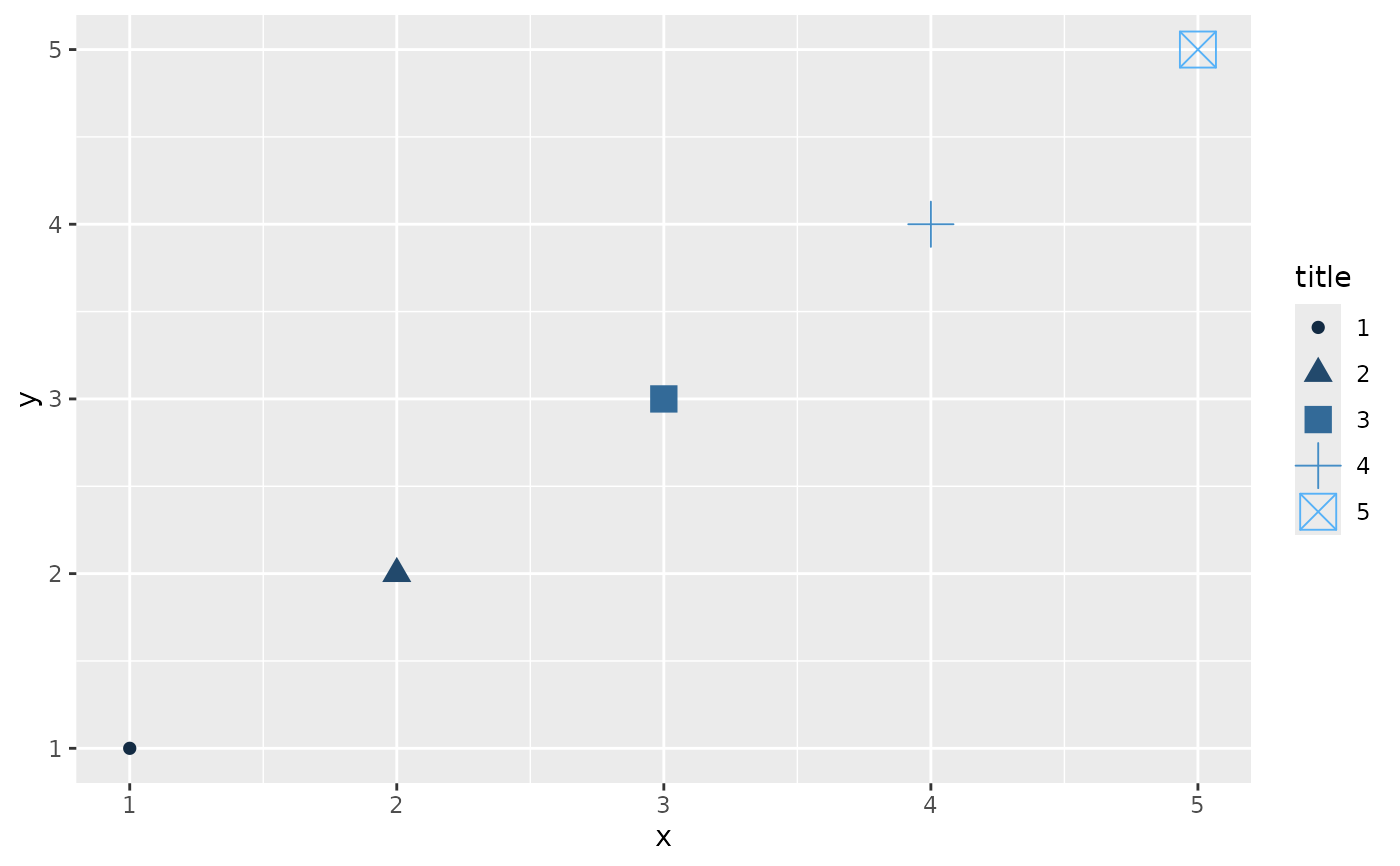# same as
g <- guide_legend("title")
p + guides(colour = g, size = g, shape = g)
#> Warning: Using size for a discrete variable is not advised.p + theme(legend.position = "bottom")
#> Warning: Using size for a discrete variable is not advised.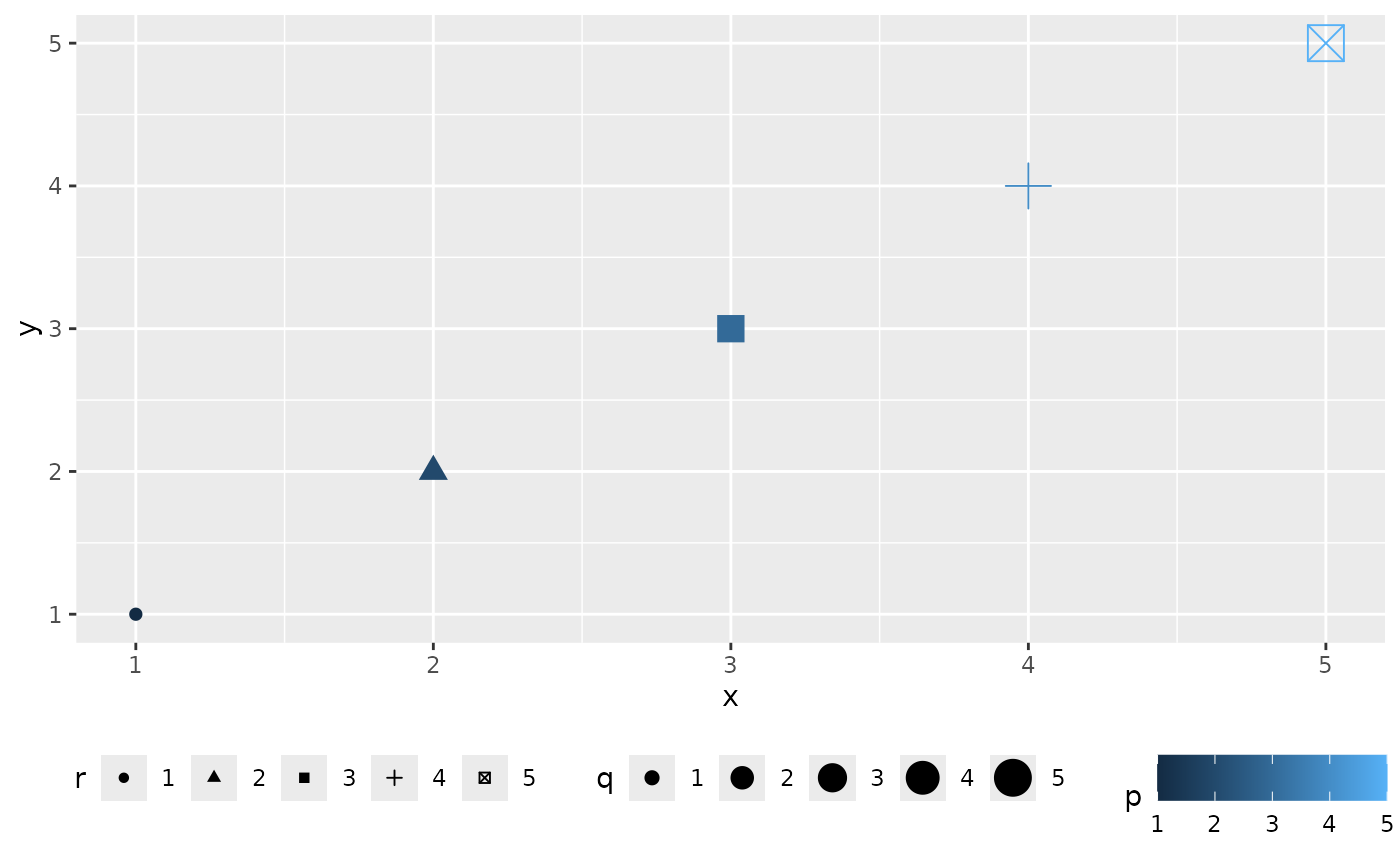# position of guides

# Set order for multiple guides
ggplot(mpg, aes(displ, cty)) +
geom_point(aes(size = hwy, colour = cyl, shape = drv)) +
guides(
colour = guide_colourbar(order = 1),
shape = guide_legend(order = 2),
size = guide_legend(order = 3)
)# }